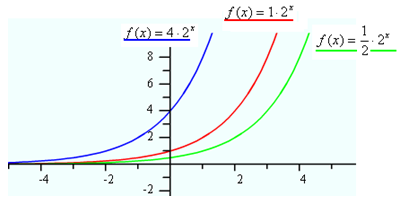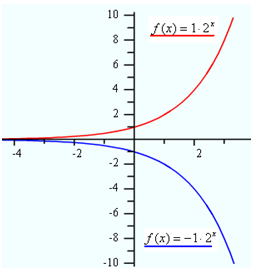Exponential Curves
Changing a

Now consider the effect that the value of a has on the exponential curve. The value of a determines where the curve crosses the y-axis. The graph below displays the curves of three exponential functions with the same base, but with varying positive values of a. Notice that the y-intercept is the same value as a and that the overall shape of the curve does not change. When a is positive the curve will increase from left to right.What happens to the curve when a is negative? The following graph demonstrates the effect of a negative value for a. The curve still intersects the y-axis at the value of a, but the curve has now been reflected across the x-axis and the function decreases from left to right.